## Direct Mapping-

Before you go through this article, make sure that you have gone through the previous article on Direct Mapping.

In direct mapping,

• A particular block of main memory can be mapped to one particular cache line only.
• Block ‘j’ of main memory will map to line number (j mod number of cache lines) of the cache.
• There is no need of any replacement algorithm.

In this article, we will discuss practice problems based on direct mapping.

## Problem-01:

Consider a direct mapped cache of size 16 KB with block size 256 bytes. The size of main memory is 128 KB. Find-

1. Number of bits in tag
2. Tag directory size

## Solution-

Given-

• Cache memory size = 16 KB
• Block size = Frame size = Line size = 256 bytes
• Main memory size = 128 KB

We consider that the memory is byte addressable.

### Number of Bits in Physical Address-

We have,

Size of main memory

= 128 KB

= 217 bytes

Thus, Number of bits in physical address = 17 bits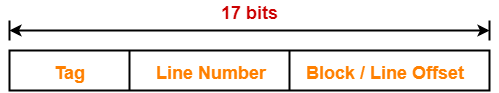### Number of Bits in Block Offset-

We have,

Block size

= 256 bytes

= 28 bytes

Thus, Number of bits in block offset = 8 bits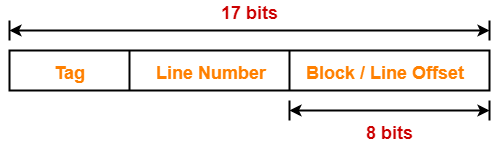### Number of Bits in Line Number-

Total number of lines in cache

= Cache size / Line size

= 16 KB / 256 bytes

= 214 bytes / 28 bytes

= 26 lines

Thus, Number of bits in line number = 6 bits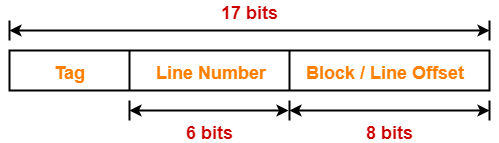### Number of Bits in Tag-

Number of bits in tag

= Number of bits in physical address – (Number of bits in line number + Number of bits in block offset)

= 17 bits – (6 bits + 8 bits)

= 17 bits – 14 bits

= 3 bits

Thus, Number of bits in tag = 3 bits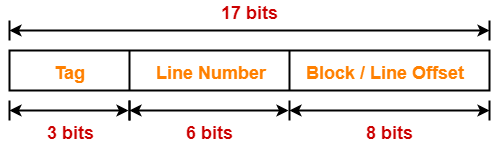### Tag Directory Size-

Tag directory size

= Number of tags x Tag size

= Number of lines in cache x Number of bits in tag

= 26 x 3 bits

= 192 bits

= 24 bytes

Thus, size of tag directory = 24 bytes

## Problem-02:

Consider a direct mapped cache of size 512 KB with block size 1 KB. There are 7 bits in the tag. Find-

1. Size of main memory
2. Tag directory size

## Solution-

Given-

• Cache memory size = 512 KB
• Block size = Frame size = Line size = 1 KB
• Number of bits in tag = 7 bits

We consider that the memory is byte addressable.

### Number of Bits in Block Offset-

We have,

Block size

= 1 KB

= 210 bytes

Thus, Number of bits in block offset = 10 bits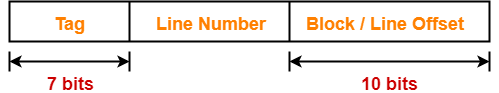### Number of Bits in Line Number-

Total number of lines in cache

= Cache size / Line size

= 512 KB / 1 KB

= 29 lines

Thus, Number of bits in line number = 9 bits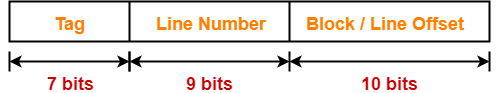### Number of Bits in Physical Address-

Number of bits in physical address

= Number of bits in tag + Number of bits in line number + Number of bits in block offset

= 7 bits + 9 bits + 10 bits

= 26 bits

Thus, Number of bits in physical address = 26 bits

### Size of Main Memory-

We have,

Number of bits in physical address = 26 bits

Thus, Size of main memory

= 226 bytes

= 64 MB

### Tag Directory Size-

Tag directory size

= Number of tags x Tag size

= Number of lines in cache x Number of bits in tag

= 29 x 7 bits

= 3584 bits

= 448 bytes

Thus, size of tag directory = 448 bytes

## Problem-03:

Consider a direct mapped cache with block size 4 KB. The size of main memory is 16 GB and there are 10 bits in the tag. Find-

1. Size of cache memory
2. Tag directory size

## Solution-

Given-

• Block size = Frame size = Line size = 4 KB
• Size of main memory = 16 GB
• Number of bits in tag = 10 bits

We consider that the memory is byte addressable.

### Number of Bits in Physical Address-

We have,

Size of main memory

= 16 GB

= 234 bytes

Thus, Number of bits in physical address = 34 bits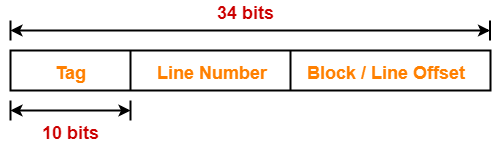### Number of Bits in Block Offset-

We have,

Block size

= 4 KB

= 212 bytes

Thus, Number of bits in block offset = 12 bits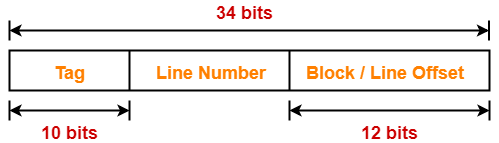### Number of Bits in Line Number-

Number of bits in line number

= Number of bits in physical address – (Number of bits in tag + Number of bits in block offset)

= 34 bits – (10 bits + 12 bits)

= 34 bits – 22 bits

= 12 bits

Thus, Number of bits in line number = 12 bits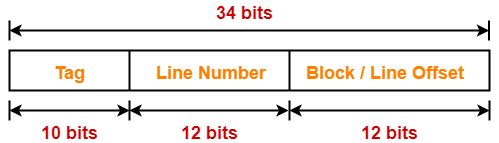### Number of Lines in Cache-

We have-

Number of bits in line number = 12 bits

Thus, Total number of lines in cache = 212 lines

### Size of Cache Memory-

Size of cache memory

= Total number of lines in cache x Line size

= 212 x 4 KB

= 214 KB

= 16 MB

Thus, Size of cache memory = 16 MB

### Tag Directory Size-

Tag directory size

= Number of tags x Tag size

= Number of lines in cache x Number of bits in tag

= 212 x 10 bits

= 40960 bits

= 5120 bytes

Thus, size of tag directory = 5120 bytes

## Problem-04:

Consider a direct mapped cache of size 32 KB with block size 32 bytes. The CPU generates 32 bit addresses. The number of bits needed for cache indexing and the number of tag bits are respectively-

1. 10, 17
2. 10, 22
3. 15, 17
4. 5, 17

## Solution-

Given-

• Cache memory size = 32 KB
• Block size = Frame size = Line size = 32 bytes
• Number of bits in physical address = 32 bits

### Number of Bits in Block Offset-

We have,

Block size

= 32 bytes

= 25 bytes

Thus, Number of bits in block offset = 5 bits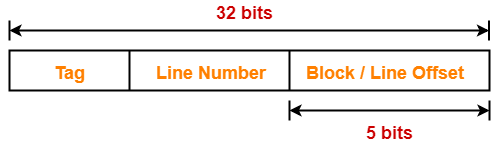### Number of Bits in Line Number-

Total number of lines in cache

= Cache size / Line size

= 32 KB / 32 bytes

= 210 lines

Thus, Number of bits in line number = 10 bits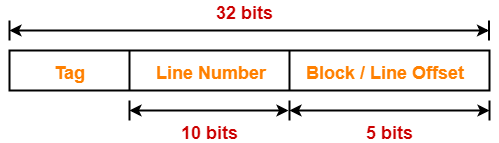### Number of Bits Required For Cache Indexing-

Number of bits required for cache indexing

= Number of bits in line number

= 10 bits

### Number Of Bits in Tag-

Number of bits in tag

= Number of bits in physical address – (Number of bits in line number + Number of bits in block offset)

= 32 bits – (10 bits + 5 bits)

= 32 bits – 15 bits

= 17 bits

Thus, Number of bits in tag = 17 bits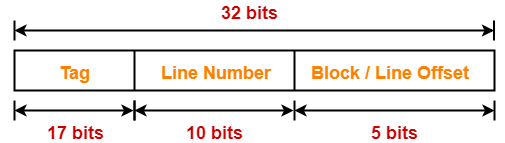Thus, Option (A) is correct.

## Problem-05:

Consider a machine with a byte addressable main memory of 232 bytes divided into blocks of size 32 bytes. Assume that a direct mapped cache having 512 cache lines is used with this machine. The size of the tag field in bits is ______.

## Solution-

Given-

• Main memory size = 232 bytes
• Block size = Frame size = Line size = 32 bytes
• Number of lines in cache = 512 lines

### Number of Bits in Physical Address-

We have,

Size of main memory

= 232 bytes

Thus, Number of bits in physical address = 32 bits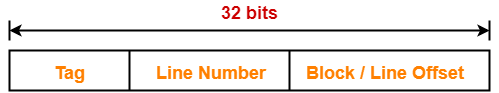### Number of Bits in Block Offset-

We have,

Block size

= 32 bytes

= 25 bytes

Thus, Number of bits in block offset = 5 bits### Number of Bits in Line Number-

Total number of lines in cache

= 512 lines

= 29 lines

Thus, Number of bits in line number = 9 bits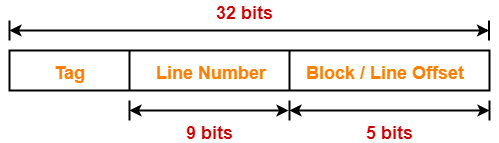### Number Of Bits in Tag-

Number of bits in tag

= Number of bits in physical address – (Number of bits in line number + Number of bits in block offset)

= 32 bits – (9 bits + 5 bits)

= 32 bits – 14 bits

= 18 bits

Thus, Number of bits in tag = 18 bits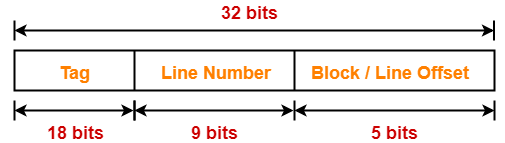## Problem-06:

An 8 KB direct-mapped write back cache is organized as multiple blocks, each of size 32 bytes. The processor generates 32 bit addresses. The cache controller maintains the tag information for each cache block comprising of the following-

• 1 valid bit
• 1 modified bit
• As many bits as the minimum needed to identify the memory block mapped in the cache

What is the total size of memory needed at the cache controller to store meta data (tags) for the cache?

1. 4864 bits
2. 6144 bits
3. 6656 bits
4. 5376 bits

## Solution-

Given-

• Cache memory size = 8 KB
• Block size = Frame size = Line size = 32 bytes
• Number of bits in physical address = 32 bits

### Number of Bits in Block Offset-

We have,

Block size

= 32 bytes

= 25 bytes

Thus, Number of bits in block offset = 5 bits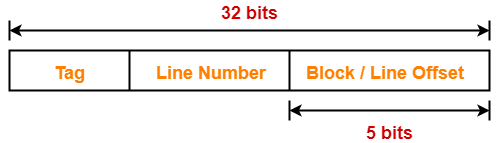### Number of Bits in Line Number-

Total number of lines in cache

= Cache memory size / Line size

= 8 KB / 32 bytes

= 213 bytes / 25 bytes

= 28 lines

Thus, Number of bits in line number = 8 bits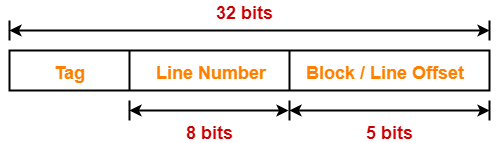### Number Of Bits in Tag-

Number of bits in tag

= Number of bits in physical address – (Number of bits in line number + Number of bits in block offset)

= 32 bits – (8 bits + 5 bits)

= 32 bits – 13 bits

= 19 bits

Thus, Number of bits in tag = 19 bits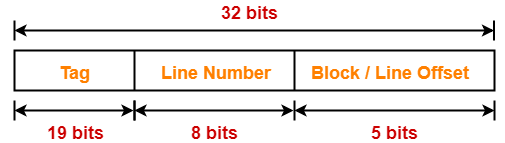### Memory Size Needed At Cache Controller-

Size of memory needed at cache controller

= Number of lines in cache x (1 valid bit + 1 modified bit + 19 bits to identify block)

= 28 x 21 bits

= 5376 bits

To watch video solutions and practice more problems,

Watch this Video Lecture

Next Article- Practice Problems On Fully Associative Mapping

Get more notes and other study material of Computer Organization and Architecture.

Watch video lectures by visiting our YouTube channel LearnVidFun.

## Cache Mapping-

Before you go through this article, make sure that you have gone through the previous article on Cache Mapping.

 Cache mapping is a technique by which the contents of main memory are brought into the cache memory.

Different cache mapping techniques are-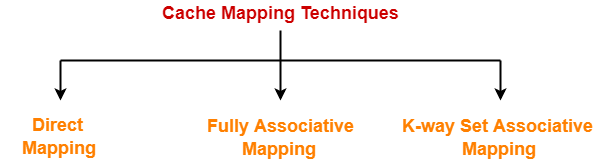1. Direct Mapping
2. Fully Associative Mapping
3. K-way Set Associative Mapping

## Direct Mapping-

In direct mapping,

• A particular block of main memory can map to only one particular line of the cache.
• The line number of cache to which a particular block can map is given by-

 Cache line number= ( Main Memory Block Address ) Modulo (Number of lines in Cache)

In direct mapping, the physical address is divided as-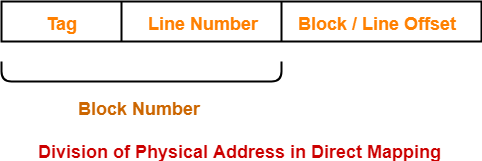## Direct Mapped Cache-

 Direct mapped cache employs direct cache mapping technique.

The following steps explain the working of direct mapped cache-

After CPU generates a memory request,

• The line number field of the address is used to access the particular line of the cache.
• The tag field of the CPU address is then compared with the tag of the line.
• If the two tags match, a cache hit occurs and the desired word is found in the cache.
• If the two tags do not match, a cache miss occurs.
• In case of a cache miss, the required word has to be brought from the main memory.
• It is then stored in the cache together with the new tag replacing the previous one.

## Implementation-

The following diagram shows the implementation of direct mapped cache-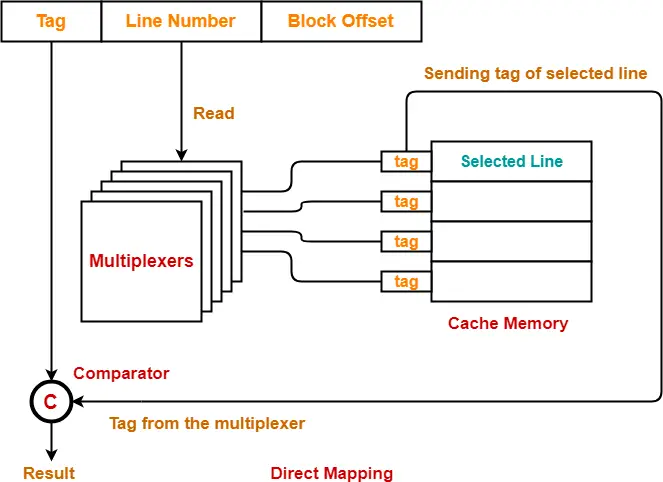(For simplicity, this diagram shows does not show all the lines of multiplexers)

The steps involved are as follows-

## Step-01:

• Each multiplexer reads the line number from the generated physical address using its select lines in parallel.
• To read the line number of L bits, number of select lines each multiplexer must have = L.

## Step-02:

• After reading the line number, each multiplexer goes to the corresponding line in the cache memory using its input lines in parallel.
• Number of input lines each multiplexer must have = Number of lines in the cache memory

## Step-03:

• Each multiplexer outputs the tag bit it has selected from that line to the comparator using its output line.
• Number of output line in each multiplexer = 1.

## UNDERSTAND

It is important to understand-

• A multiplexer can output only a single bit on output line.
• So, to output the complete tag to the comparator,

Number of multiplexers required = Number of bits in the tag

• Each multiplexer is configured to read the tag bit at specific location.

## Example-

• 1st multiplexer is configured to output the first bit of the tag.
• 2nd multiplexer is configured to output the second bit of the tag.
• 3rd multiplexer is configured to output the third bit of the tag and so on.

So,

• Each multiplexer selects the tag bit of the selected line for which it has been configured and outputs on the output line.
• The complete tag as a whole is sent to the comparator for comparison in parallel.

## Step-04:

• Comparator compares the tag coming from the multiplexers with the tag of the generated address.
• Only one comparator is required for the comparison where-

Size of comparator = Number of bits in the tag

• If the two tags match, a cache hit occurs otherwise a cache miss occurs.

## Hit latency-

The time taken to find out whether the required word is present in the Cache Memory or not is called as hit latency.

For direct mapped cache,

 Hit latency = Multiplexer latency + Comparator latency

## Important Results-

Following are the few important results for direct mapped cache-

• Block j of main memory can map to line number (j mod number of lines in cache) only of the cache.
• Number of multiplexers required = Number of bits in the tag
• Size of each multiplexer = Number of lines in cache x 1
• Number of comparators required = 1
• Size of comparator = Number of bits in the tag
• Hit latency = Multiplexer latency + Comparator latency

To gain better understanding about direct mapping,

Watch this Video Lecture

Next Article- Practice Problems On Direct Mapping

Get more notes and other study material of Computer Organization and Architecture.

Watch video lectures by visiting our YouTube channel LearnVidFun.